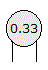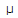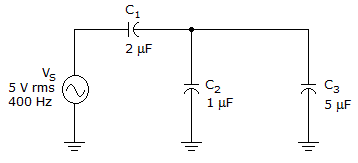# Electronics - Capacitors

### Exercise :: Capacitors - Filling the Blanks

11.

A ___ capacitor allows ac signals to pass while blocking dc signals.

 A. filter B. bypass C. decoupling D. coupling

Explanation:

No answer description available for this question. Let us discuss.

12.

The rate of charge or discharge of a capacitor is based on ___ function.

 A. an exponential B. a linear C. a parabolic D. a trigonometric

Explanation:

No answer description available for this question. Let us discuss.

13.The given image is a _____ capacitor.

 A. 0.33 pF B. 0.00033F C. 33,000 pF D. 0.33F

Explanation:

No answer description available for this question. Let us discuss.

14.The reactance of C2 is _____ in the given circuit.

 A. 66B. 80C. 199D. 398